Courses

# Test: Patterns - 2

## 20 Questions MCQ Test Mathematics (Maths) for Class 2 | Test: Patterns - 2

Description
This mock test of Test: Patterns - 2 for Class 2 helps you for every Class 2 entrance exam. This contains 20 Multiple Choice Questions for Class 2 Test: Patterns - 2 (mcq) to study with solutions a complete question bank. The solved questions answers in this Test: Patterns - 2 quiz give you a good mix of easy questions and tough questions. Class 2 students definitely take this Test: Patterns - 2 exercise for a better result in the exam. You can find other Test: Patterns - 2 extra questions, long questions & short questions for Class 2 on EduRev as well by searching above.
QUESTION: 1

### Which of the following numbers has 3 in tens place?

Solution:

Of the given numbers, 438 has 3 in tens place.

QUESTION: 2

### Find the missing number in the following.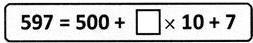Solution:

597 = 500 + 90 + 7 = 500 + 9 × 10 + 7

QUESTION: 3

### Who is holding an object with an even number on it?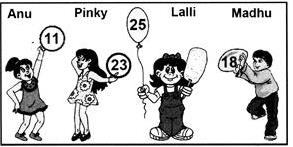Solution:

Madhu, since 18 is an even number.

QUESTION: 4

Which of the following is the predecessor of 390?

Solution:
QUESTION: 5

Which of the given statements is true about the numbers in the box?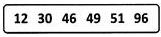Solution:

The given numbers are all between 10 and 100.

QUESTION: 6

Identify the number having ?3? in the hundreds place, ?5? in the ones place and ?2? in the tens place.

Solution: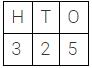QUESTION: 7

The numbers from 1 to 25 are written one beside the other. Which digit is in the 25th place?

Solution: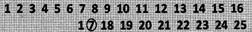QUESTION: 8

Which is the greatest even number formed by using the digits 3, 2 and 1 only once?

Solution:
QUESTION: 9

Which is the sum of the place values of 4 and 2 in 234?

Solution:

Place value of 2 = 200 Place value of 4 = 4 Sum = 200 + 4 = 204

QUESTION: 10

How can seventy nine be written in numerals?

Solution:

79 = 7 × 10 + 9 × 1 = 70 + 9

QUESTION: 11

Which number replaces the question mark in the following?
849 ? 851

Solution:

849 850 851

QUESTION: 12

Satish has collected 387 stamps. Nearly how many stamps does Satish have?

Solution: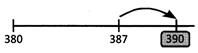QUESTION: 13

Which number has the greatest value in the hundreds place?

Solution: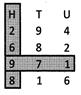QUESTION: 14

Which is the largest three-digit number?

Solution:

The largest 3 - digit number is 999.

QUESTION: 15

How many zeros are there in ten hundreds?

Solution:

10 × 100 = 1000 which has 3 zeroes.

QUESTION: 16

Look at the number line given.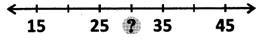Which number replaces the question mark on it?

Solution:

25 + 5 = 30

QUESTION: 17

How many hundreds are there in 843?

Solution:

The number 843 has 8 hundreds.

QUESTION: 18

Which of the following is the same as 30 tens?

Solution:

30 × 10 = 300 = 3 hundreds

QUESTION: 19

What is the place value of 5 in the number 215?

Solution:

The place value of 5 in 215 is 5 × 1 = 5

QUESTION: 20

How many tens make a thousand?

Solution:

100 x 10 = 1000